# NCERT Exemplar Class 8 Science Solutions for Chapter 13 - Sound

## NCERT Exemplar Solutions Class 8 Science Chapter 13 – Free PDF Download

NCERT Exemplar Solution for Class 8 Science Chapter 13 Sound is a crucial study material required for the students studying in CBSE Class 8. This exemplar has answers to the questions provided in NCERT Exemplar. By solving NCERT Exemplar Solutions students get all-important problem-solving capacity. This Exemplar solution has a different kind of questions such as MCQs, very short answer questions, short answer questions, long answer questions and numerical problems. This exemplar has answers to 10 MCQs, 5 very short questions, 5 short answer questions and, 5 long answer questions.

In this chapter, the students will learn, how sound is produced by a vibrating body, sound produced by humans, how sound propagates through, air and water. Solving the NCERT Exemplar Solutions Class 8 Science Chapter 13 Sound will help the students to understand the higher-order thinking questions as well as to score good marks in the exam. To download the NCERT Exemplar questions visit the below downloadable link.

### Download PDF of NCERT Exemplar Class 8 Science Solutions for Chapter 13 – Sound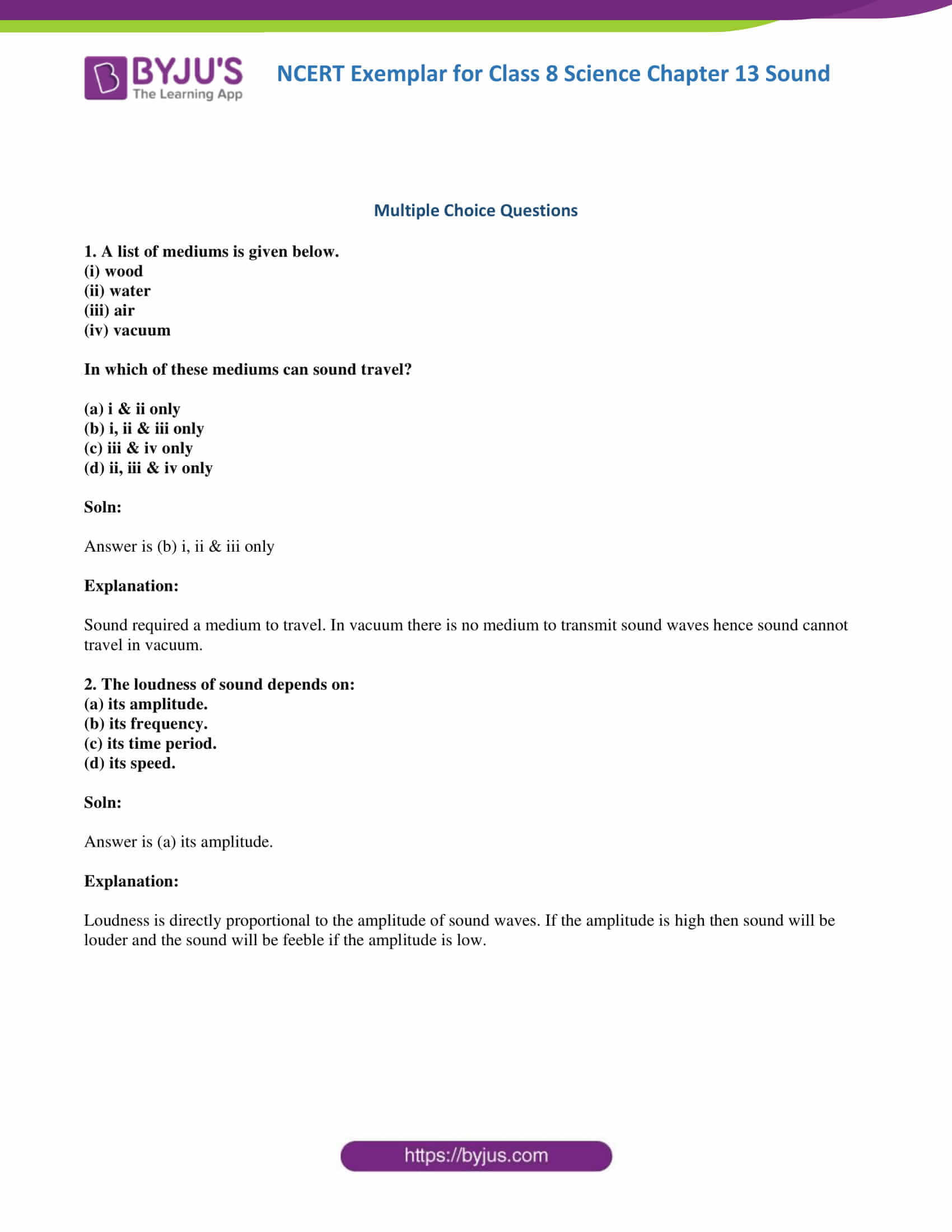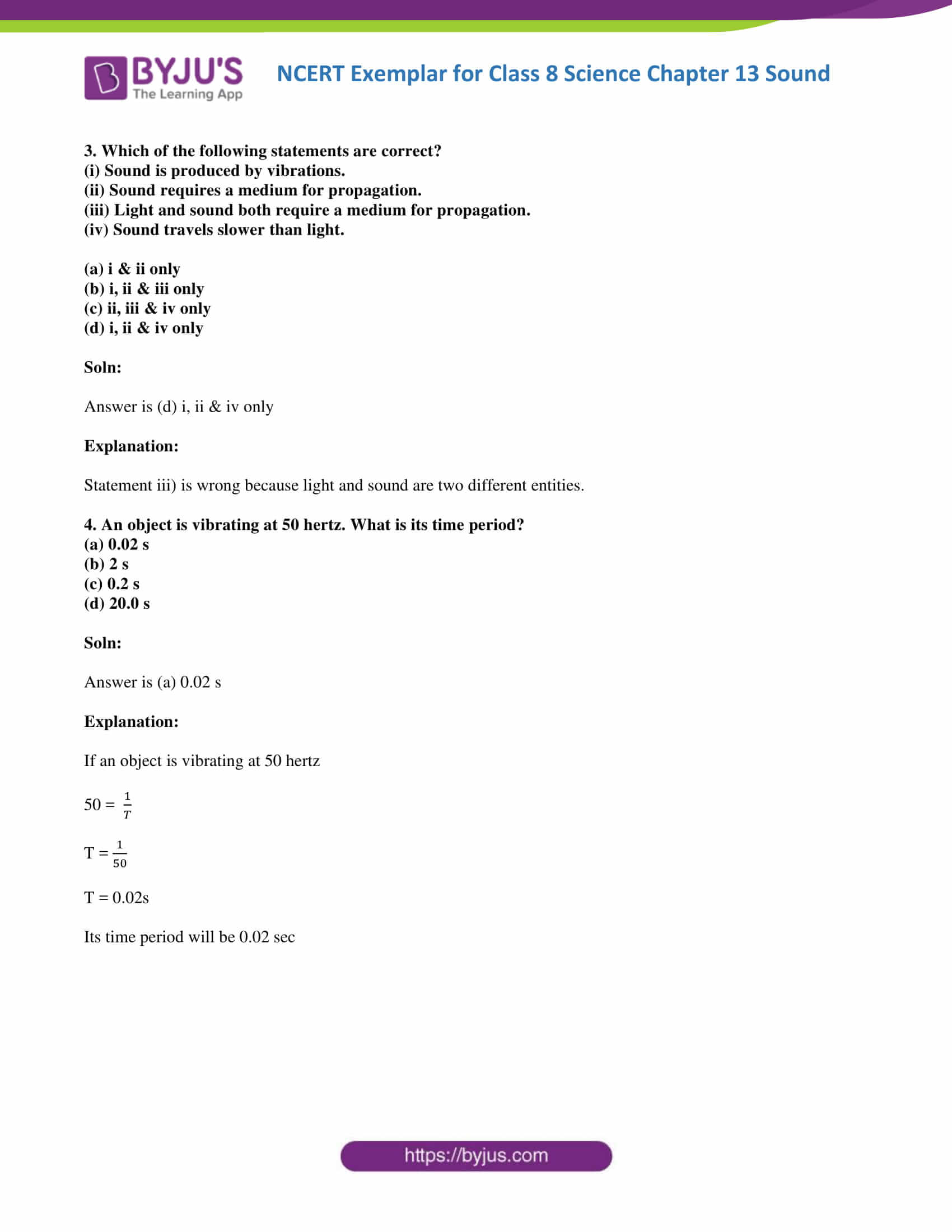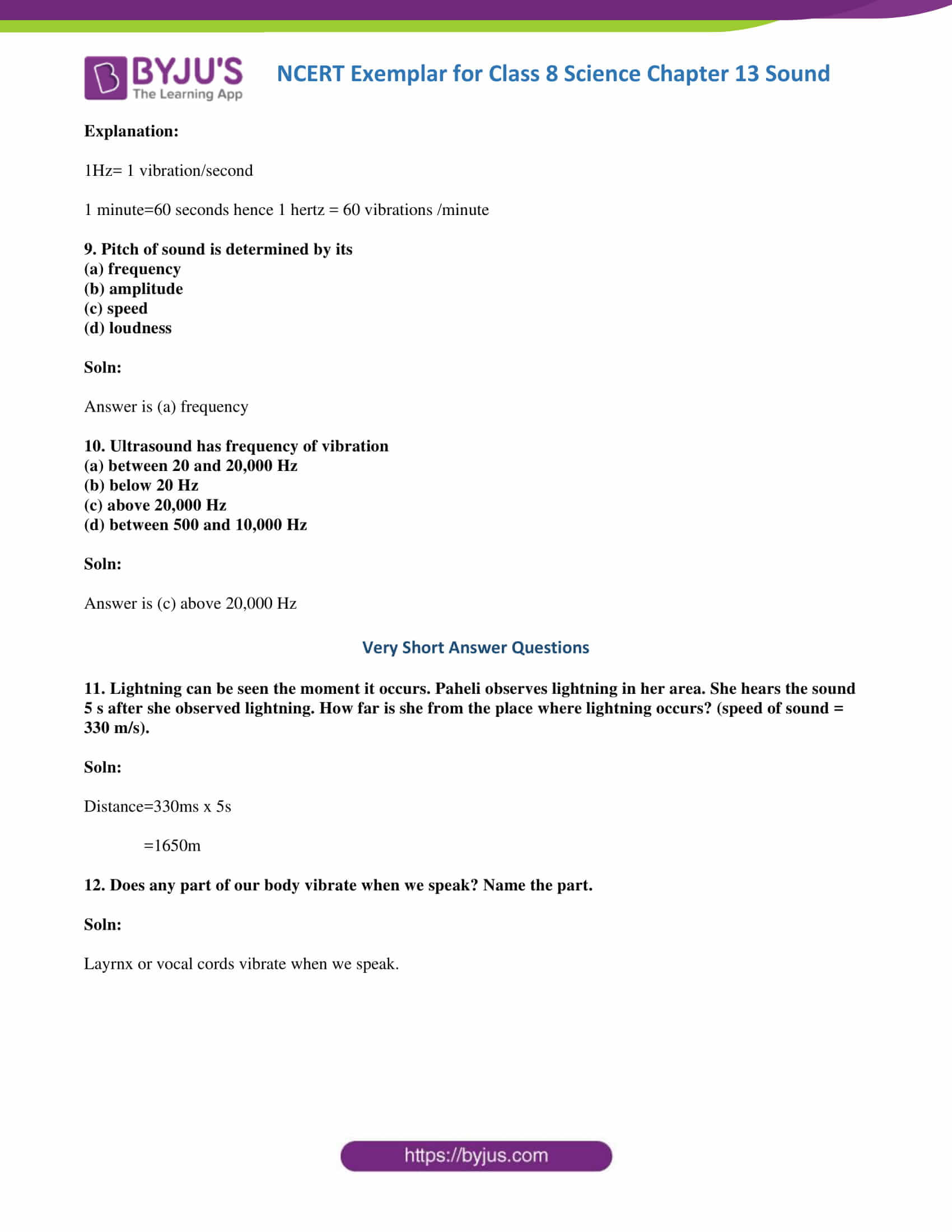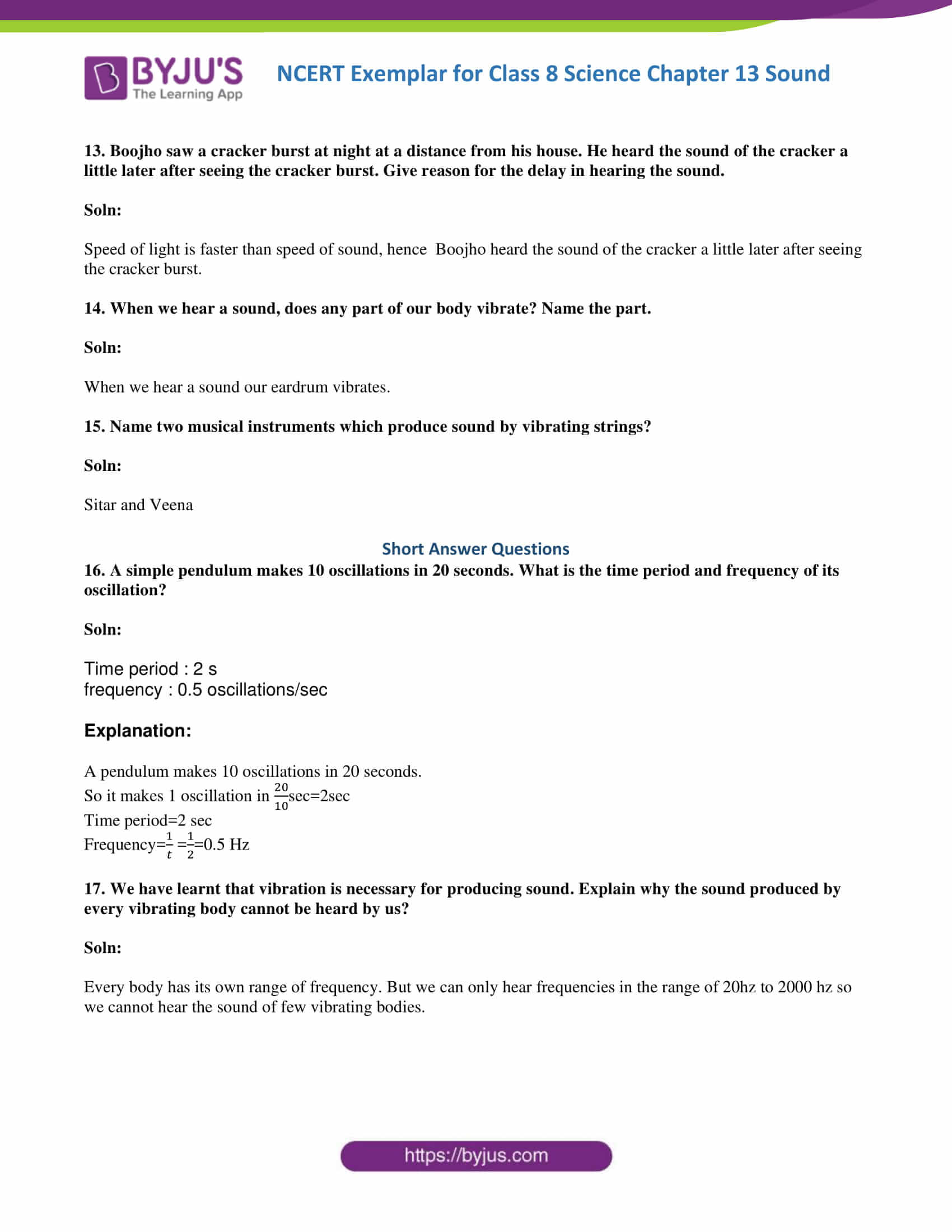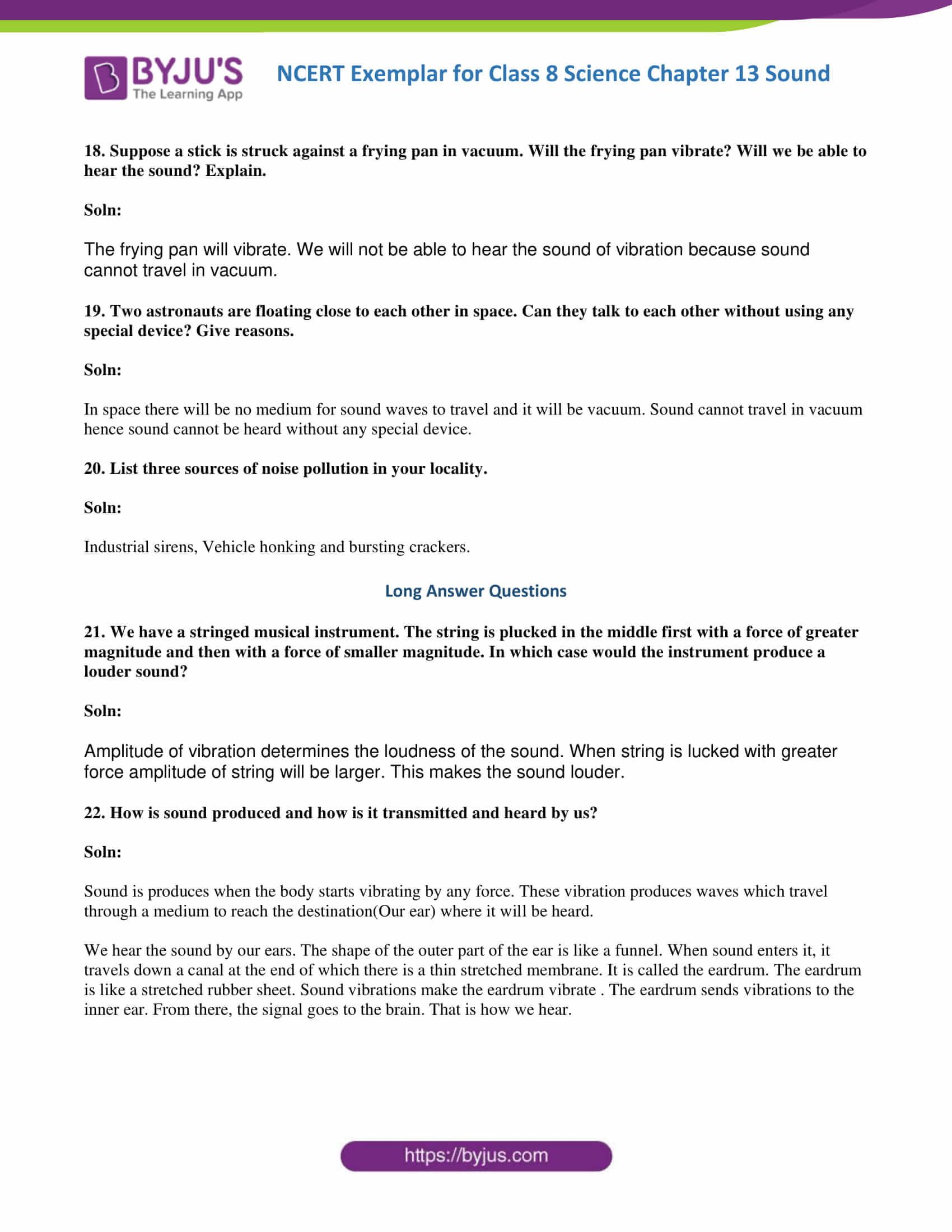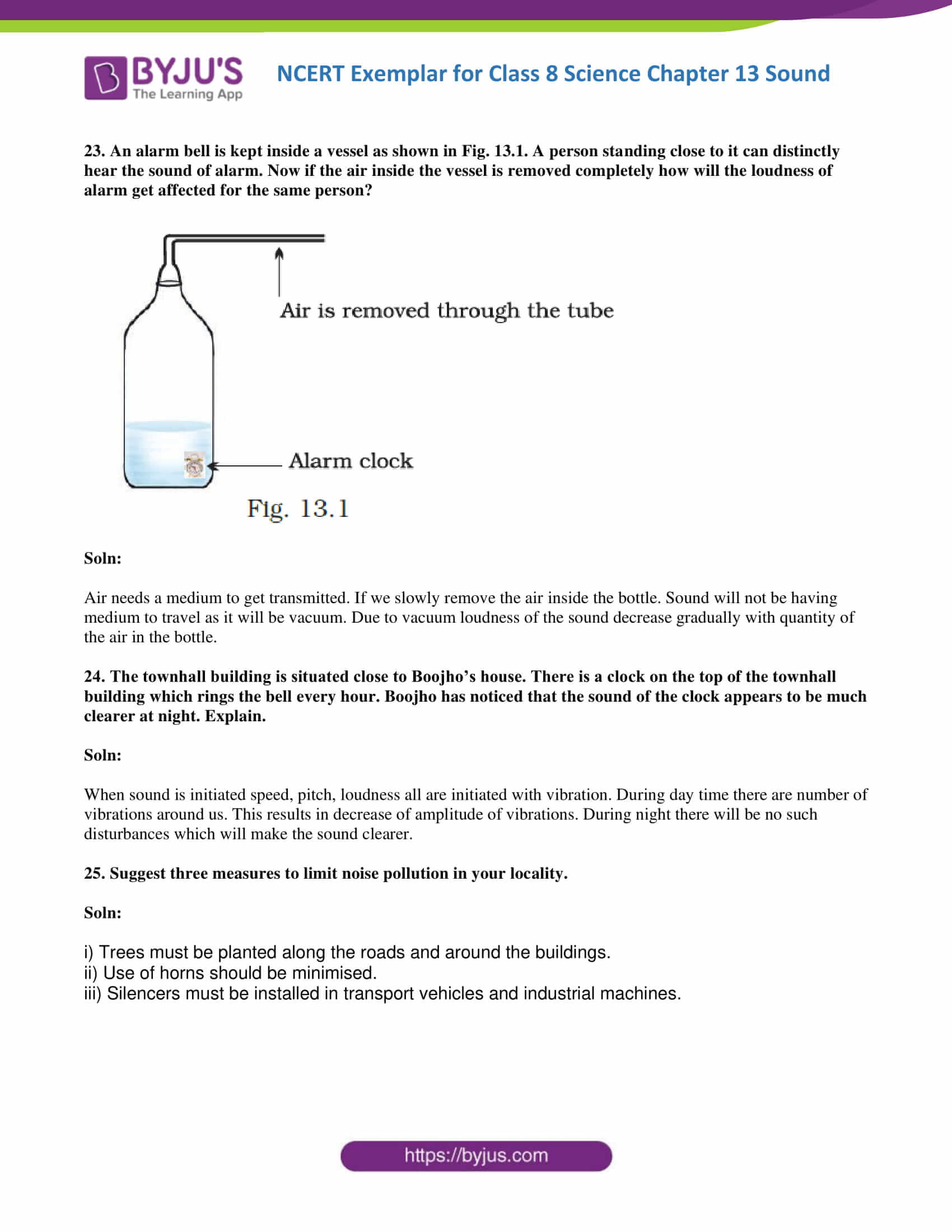### Multiple Choice Questions

1. A list of mediums is given below.

(i) wood

(ii) water

(iii) air

(iv) vacuum

In which of these mediums can sound travel?

(a) i & ii only

(b) i, ii & iii only

(c) iii & iv only

(d) ii, iii & iv only

Soln:

Answer is (b) i, ii & iii only

Explanation:

Sound required a medium to travel. In a vacuum there is no medium to transmit sound waves hence sound cannot travel in a vacuum.

2. The loudness of a sound depends on:

(a) its amplitude.

(b) its frequency.

(c) its time period.

(d) its speed.

Soln:

Explanation:

Loudness is directly proportional to the amplitude of sound waves. If the amplitude is high then the sound will be louder and the sound will be feeble if the amplitude is low.

3. Which of the following statements are correct?

(i) Sound is produced by vibrations.

(ii) Sound requires a medium for propagation.

(iii) Light and sound both require a medium for propagation.

(iv) Sound travels slower than light.

(a) i & ii only

(b) i, ii & iii only

(c) ii, iii & iv only

(d) i, ii & iv only

Soln:

Answer is (d) i, ii & iv only

Explanation:

Statement iii) is wrong because light and sound are two different entities.

4. An object is vibrating at 50 hertz. What is its time period?

(a) 0.02 s

(b) 2 s

(c) 0.2 s

(d) 20.0 s

Soln:

Explanation:

If an object is vibrating at 50 hertz

50 = 1/T

T = 1/50

T = 0.02s

Its time period will be 0.02 sec

5. In order to reduce the loudness of a sound, we have to

(a) decrease its frequency of vibration of the sound.

(b) increase its frequency of vibration of the sound.

(c) decrease its amplitude of vibration of the sound.

(d) increase its amplitude of vibration of the sound.

Soln:

Answer is (c) decrease its amplitude of vibration of the sound.

Explanation:

Sound is directly proportional to loudness of sound. Hence by decreasing amplitude loudness of the sound decreases.

6. The loudness of sound is measured in units of

(a) Decibel (dB)

(b) Hertz (Hz)

(c) Metre (m)

(d) Metre/Second (m/s)

Soln:

7. The loudness of sound is determined by the

(a) amplitude of vibration

(b) the ratio of amplitude and frequency of vibration

(c) frequency of vibration

(d) product of amplitude and frequency of vibration

Soln:

Answer is (a) amplitude of vibration

Explanation:

Loudness is directly proportional to the amplitude of sound waves. If the amplitude is high then the sound will be louder and the sound will be feeble if the amplitude is low.

8. 1 hertz is equal to

(a) 1 vibration per minute

(b) 10 vibrations per minute

(c) 60 vibrations per minute

(d) 600 vibrations per minute

Soln:

Answer is (c) 60 vibrations per minute

Explanation:

1Hz= 1 vibration/second

1 minute=60 seconds hence 1 hertz = 60 vibrations /minute

9. Pitch of sound is determined by its

(a) frequency

(b) amplitude

(c) speed

(d) loudness

Soln:

10. Ultrasound has a frequency of vibration

(a) between 20 and 20,000 Hz

(b) below 20 Hz

(c) above 20,000 Hz

(d) between 500 and 10,000 Hz

Soln:

Answer is (c) above 20,000 Hz

11. Lightning can be seen as the moment it occurs. Paheli observes lightning in her area. She hears the sound 5 s after she observed lightning. How far is she from the place where lightning occurs? (speed of sound = 330 m/s).

Soln:

Distance=330ms x 5s

=1650m

12. Does any part of our body vibrate when we speak? Name the part.

Soln:

Larynx or vocal cords vibrate when we speak.

13. Boojho saw a cracker burst at night at a distance from his house. He heard the sound of the cracker a little later after seeing the cracker burst. Give the reason for the delay in hearing the sound.

Soln:

Speed of light is faster than the speed of sound, hence Boojho heard the sound of the cracker a little later after seeing the cracker burst.

14. When we hear a sound, does any part of our body vibrate? Name the part.

Soln:

When we hear a sound our eardrum vibrates.

15. Name two musical instruments which produce sound by vibrating strings?

Soln:

Sitar and Veena

16. A simple pendulum makes 10 oscillations in 20 seconds. What is the time period and frequency of its oscillation?

Soln:

Time period: 2 s

frequency : 0.5 oscillations/sec

Explanation:

A pendulum makes 10 oscillations in 20 seconds.

So it makes 1 oscillation in

$$\begin{array}{l}\frac{20}{10}\end{array}$$
sec = 2sec

Time period=2 sec

Frequency=

$$\begin{array}{l}\frac{1}{t}\end{array}$$
=
$$\begin{array}{l}\frac{1}{2}\end{array}$$
= 0.5 Hz

17. We have learnt that vibration is necessary for producing sound. Explain why the sound produced by every vibrating body cannot be heard by us?

Soln:

Everybody has its own range of frequency. But we can only hear frequencies in the range of 20hz to 2000 Hz so we cannot hear the sound of a few vibrating bodies.

18. Suppose a stick is struck against a frying pan in a vacuum. Will the frying pan vibrate? Will we be able to hear the sound? Explain.

Soln:

The frying pan will vibrate. We will not be able to hear the sound of vibration because sound cannot travel in a vacuum.

19. Two astronauts are floating close to each other in space. Can they talk to each other without using any special device? Give reasons.

Soln:

In space there will be no medium for sound waves to travel and it will be the vacuum. Sound cannot travel in vacuum hence sound cannot be heard without any special device.

20. List three sources of noise pollution in your locality.

Soln:

Industrial sirens, Vehicle honking and bursting crackers.

21. We have a stringed musical instrument. The string is plucked in the middle first with a force of greater magnitude and then with a force of smaller magnitude. In which case would the instrument produce a louder sound?

Soln:

The amplitude of vibration determines the loudness of the sound. When a string is plucked with greater force amplitude of string will be larger. This makes the sound louder.

22. How is the sound produced and how is it transmitted and heard by us?

Soln:

Sound is produced when the body starts vibrating by any force. This vibration produces waves which travel through a medium to reach the destination(Our ear) where it will be heard.

We hear the sound by our ears. The shape of the outer part of the ear is like a funnel. When sound enters it, it travels down a canal at the end of which there is a thin stretched membrane. It is called the eardrum. The eardrum is like a stretched rubber sheet. Sound vibrations make the eardrum vibrate. The eardrum sends vibrations to the inner ear. From there, the signal goes to the brain. That is how we hear.

23. An alarm bell is kept inside a vessel as shown in Fig. 13.1. A person standing close to it can distinctly hear the sound of the alarm. Now if the air inside the vessel is removed completely how will the loudness of alarm get affected for the same person?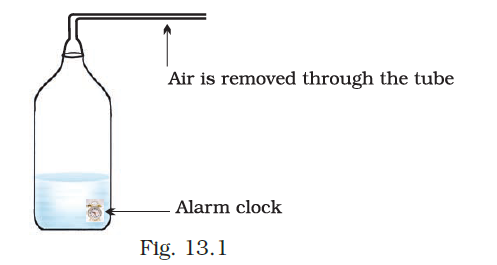Soln:

Air needs a medium to get transmitted. If we slowly remove the air inside the bottle. Sound will not be having medium to travel as it will be the vacuum. Due to vacuum loudness of the sound decrease gradually with the quantity of the air in the bottle.

24. The town hall building is situated close to Boojho’s house. There is a clock on the top of the town hall building which rings the bell every hour. Boojho has noticed that the sound of the clock appears to be much clearer at night. Explain.

Soln:

• When sound is initiated speed, pitch, loudness all are initiated with vibration. During the day time there is a number of vibrations around us. This results in a decrease of the amplitude of vibrations. During the night there will be no such disturbances which will make the sound clearer.

25. Suggest three measures to limit noise pollution in your locality.

Soln:

i) Trees must be planted along the roads and around the buildings.

ii) Use of horns should be minimised.

iii) Silencers must be installed in transport vehicles and industrial machines.

### Important topics covered in NCERT Exemplar Solutions for Class 8 Science Chapter 13 Sound

1. The sound is Produced by a Vibrating Body
2. Sound Produced by Humans
3. Sound Needs a Medium of Propagation
4. We Hear Sound through Our Ears
5. Amplitude, Time Period and Frequency of a Vibration
6. Audible and Inaudible Sounds
7. Noise and Music
8. Noise Pollution.

Students are suggested to visit the website for the latest NCERT Solutions, NCERT Exemplar, notes, sample papers and previous year question papers for Class 6 to 12 and get full assistance for the exam. Students can also download BYJU’S The Learning App to study using interactive and engaging videos.

## Frequently Asked Questions on NCERT Exemplar Solutions for Class 8 Science Chapter 13

### What are the topics and subtopics present in the Chapter 13 of NCERT Exemplar Solutions for Class 8 Science?

The topics and subtopics present in the Chapter 13 of NCERT Exemplar Solutions for Class 8 Science are –
1. The sound is Produced by a Vibrating Body
2. Sound Produced by Humans
3. Sound Needs a Medium of Propagation
4. We Hear Sound through Our Ears
5. Amplitude, Time Period and Frequency of a Vibration
6. Audible and Inaudible Sounds
7. Noise and Music
8. Noise Pollution.

### How can I score full marks in the Chapter 13 of NCERT Exemplar Solutions for Class 8 Science?

After completing extensive research on each idea, the NCERT Exemplar Solutions for Class 8 Science Chapter 13 are created by highly experienced academics. Students can use these solutions to acquire a general sense of the concepts covered in this chapter while working on the textbook questions. Students can access the solutions both online and offline, depending on their needs. On BYJU’S, solutions are provided in PDF format, which students can download and use to get their questions answered quickly.

### How is the sound produced covered in the Chapter 13 of NCERT Exemplar Solutions for Class 8 Science.

Sound is produced when the body starts vibrating by any force. This vibration produces waves which travel through a medium to reach the destination(Our ear) where it will be heard. Students of Class 8 are advised to refer to the NCERT Exemplar Solutions from BYJU’S to gain a grip on the important concepts. The solutions are prepared with the main aim of helping students with their exam preparation. The stepwise explanations in a simple language boosts the confidence among students to appear for the exam without fear.